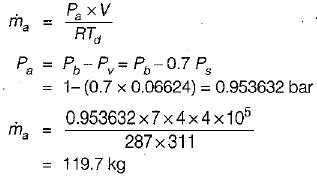Courses

# Test: Properties of Moist Air - 3

## 10 Questions MCQ Test Topicwise Question Bank for Mechanical Engineering | Test: Properties of Moist Air - 3

Description
This mock test of Test: Properties of Moist Air - 3 for Mechanical Engineering helps you for every Mechanical Engineering entrance exam. This contains 10 Multiple Choice Questions for Mechanical Engineering Test: Properties of Moist Air - 3 (mcq) to study with solutions a complete question bank. The solved questions answers in this Test: Properties of Moist Air - 3 quiz give you a good mix of easy questions and tough questions. Mechanical Engineering students definitely take this Test: Properties of Moist Air - 3 exercise for a better result in the exam. You can find other Test: Properties of Moist Air - 3 extra questions, long questions & short questions for Mechanical Engineering on EduRev as well by searching above.
QUESTION: 1

Solution:
QUESTION: 2

### For completely dry air total heat is

Solution:

For completely dry air, since latent part will not be present hence total heat will be equal to total sensible heat.

QUESTION: 3

### If two streams of air containing m1 and m2 mass of dry air and enthalpy h1 and h2 are mixed adiabaticaily then

Solution: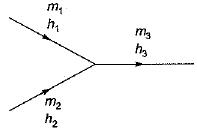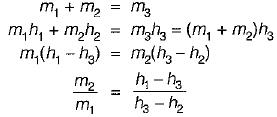QUESTION: 4

The barometer pressure is 1.03 bar, the partial pressure of air is 1 bar and the saturation partial pressure of water vapour at the same dry bulb temperature is 0.05 bar. What is the relative humidity?

Solution:

Barometer pressure, P = 1.03 bar
Pvs = 0.05 bar; Pa = 1 bar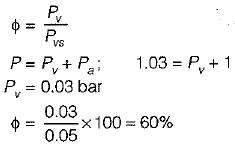QUESTION: 5

Air at state 1 (DPT = 10°C, w = 0.0040 kg/kg) mixes with air at state 2 (DPT = 18°C, w = 0.0051 kg/kg) in the ratio 1 to 3 by weight. The degree of saturation (%) of the mixture is (The specific humidity of saturated air at 13.6°C. w = 0.01 kg/kg)

Solution:

kg of moisture actually contained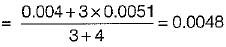kg of moisture in saturated air of mixture
= 0.01 kg/kg of air
Degree of saturation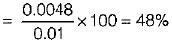QUESTION: 6

For air with a relative humidity of 80%

Solution:

For unsaturated air
DBT > WBT > DPT

QUESTION: 7

For a typical sample of ambient air (at 30°C, 75% relative humidity and standard atmospheric pressure), the amount of moisture in kg per kg of dry air will be approximately

Solution:
QUESTION: 8

5 gm of water vapour per kg of air is removed and temperature of air after removing water vapour is 25°C DBT.
Find the relative humidity. Use following table,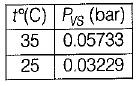Solution: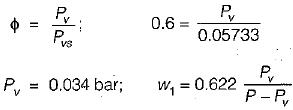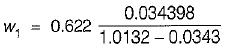w1 = 0.0218 kg vapour/kg of dry air
After removing 5 gm
w2 = 0.0168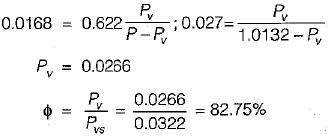QUESTION: 9

Air at state 1 (DPT = 1°C, w= 0.0040 kg/kg of da) mixes with air at state 2 (DPT = 18°C, w = 0.0051 kg/kg of da) in the ratio of 1 to 3 by weight the degree of saturation (%) of the mixture is (The specific humidity of saturated air at 13.6°C, w = 0.01 kg/kg of da)

Solution:

Moisture content in the mixture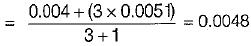kg of moisture in saturated air of mixture
= 0.01 kg/kg of air
Degree of saturation =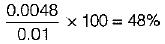QUESTION: 10

A room 7m x 4m x 4m is occupied by an air- water vapour mixture at 38°C. The atmospheric pressure is 1 bar & relative humidity is 70%. The mass of air is (take Ps at 38°C = 0.06624 bar)

Solution: